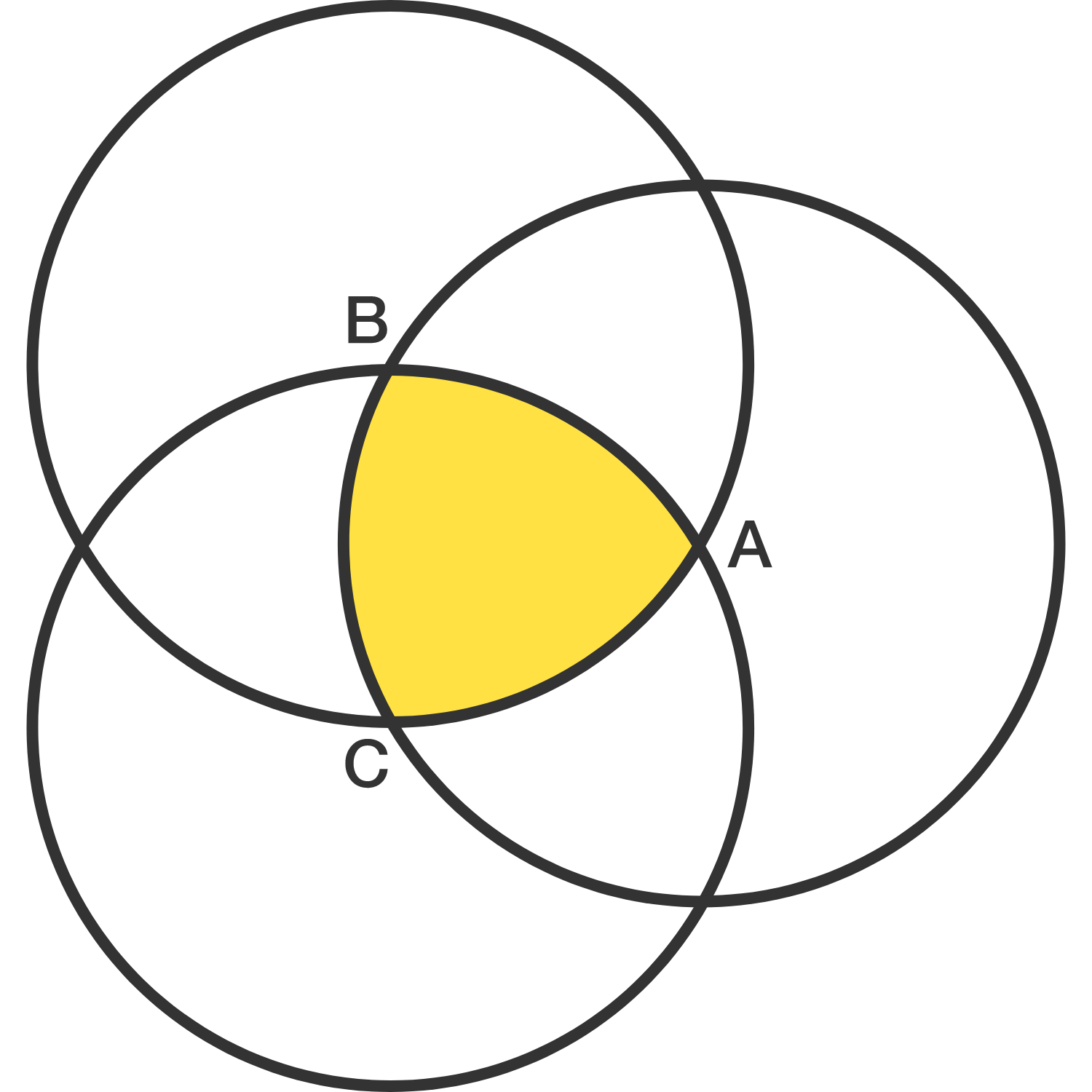# Sun of Reuleaux

Geometry Level 5A Reuleaux triangle is defined in the following way:

• The 3 apex points A, B, and C are one unit from one another
• Each pair of apex points are connected by the arc of a circle of radius 1 whose center is the third apex
• We end up with the yellow region as shown above

What is the radius of the Reuleaux triangle's circumcircle as defined by apex points A, B, and C?